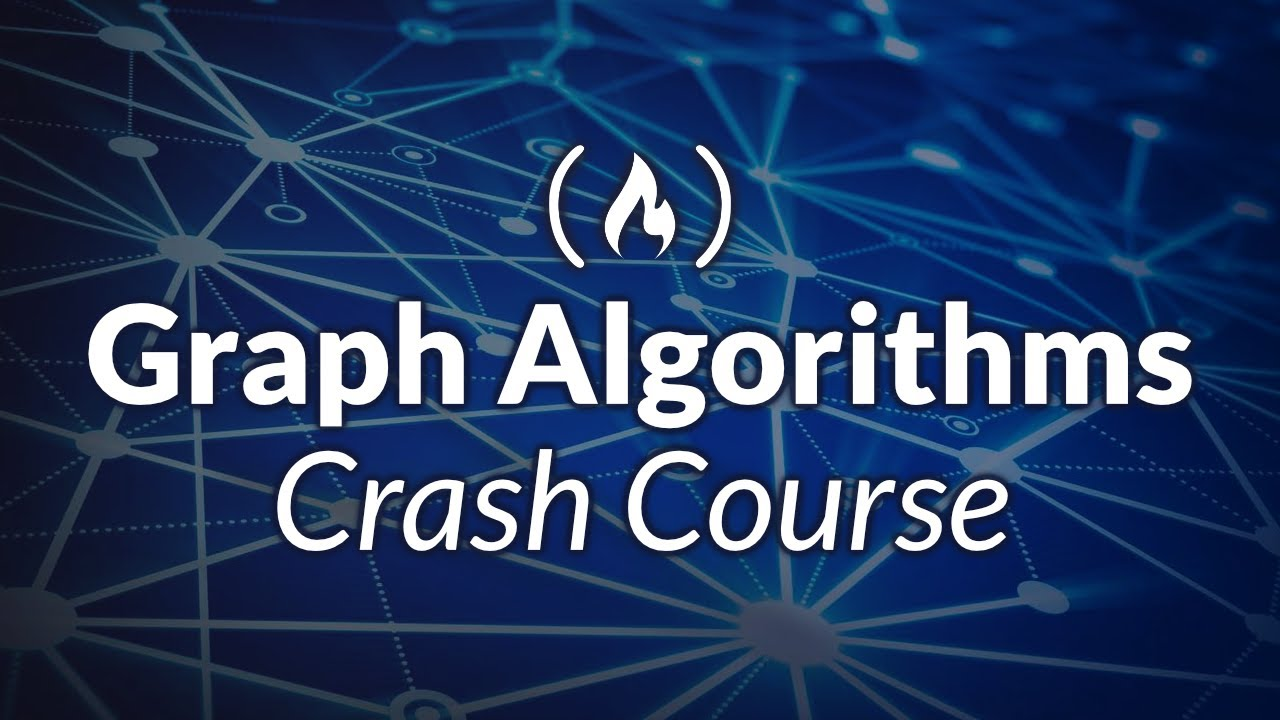# Graph Algorithms Crash Course (with Java)Learn how to use the graph data structures in this full tutorial for beginners. A Graph data structures is a non-linear data structure consisting of vertices and edges. They are used to solve many real-word problems and are commonly needed to solve coding challenges. The course uses Java.

Learn how to use the graph data structures in this full tutorial for beginners. A Graph data structures is a non-linear data structure consisting of vertices and edges. They are used to solve many real-word problems and are commonly needed to solve coding challenges. The course uses Java.

⭐️ Course Contents ⭐️
⌨️ (0:00:00) Introduction to Graphs
⌨️ (0:01:35) Graphical Explanation
⌨️ (0:03:21) Code Implementation
⌨️ (0:06:41) Vertex class
⌨️ (0:09:33) Edge class
⌨️ (0:17:46) Graph class
⌨️ (0:28:06) main method
⌨️ (0:31:31) compile and run
⌨️ (0:32:55) Introduction to Graph Traversals
⌨️ (0:34:49) Traversal Orders
⌨️ (0:35:35) DFS Traversal (Graphical Explanation)
⌨️ (0:41:56) Code Implementation of DFS
⌨️ (0:51:39) BFS Traversal (Graphical Explanation)
⌨️ (0:54:23) Code Implementation of BFS
⌨️ (1:01:09) Compile and Run
⌨️ (1:01:55) Introduction to Dijkstra’s Algorithm
⌨️ (1:02:25) Graphical Explanation
⌨️ (1:08:56) Code Implementation
⌨️ (1:12:22) Priority Queue
⌨️ (1:16:31) Iterating through the vertices
⌨️ (1:19:48) while loop
⌨️ (1:28:17) helper method
⌨️ (1:29:56) compile and run
⌨️ (1:30:21) problem occurred
⌨️ (1:30:31) shortestPathBetween()
⌨️ (1:37:27) fix to the problem
⌨️ (1:38:36) Successful Compile and Run